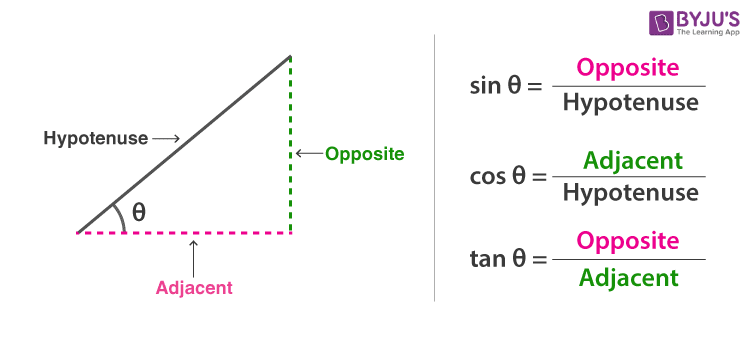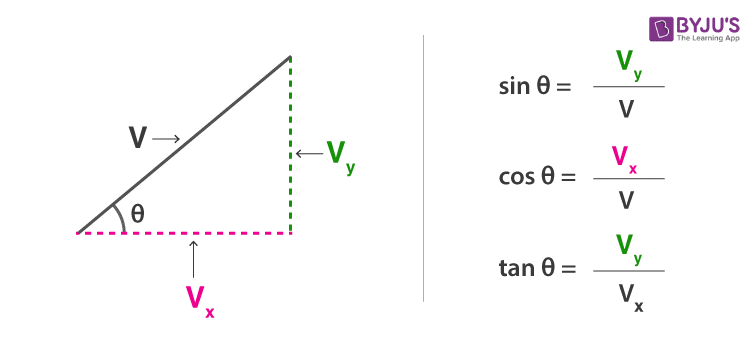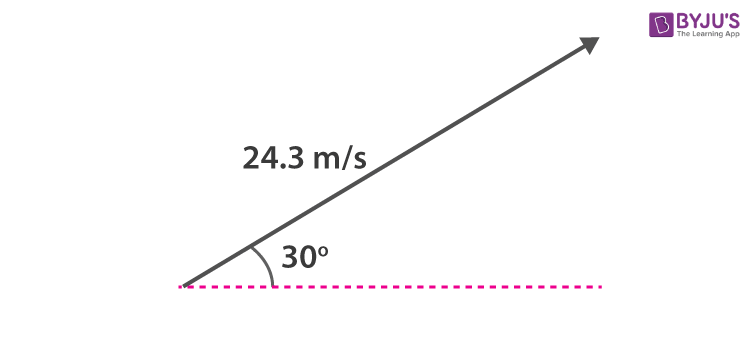# Velocity Vectors

There is no question about two-dimensional motion being more complex than one-dimensional motion since velocities can point in diagonal directions. To better understand this statement, let us consider the example of a baseball moving horizontally and vertically at the same time with a diagonal velocity v. To simplify our calculations, we break this velocity component into horizontal vx and vertical components vy.

Breaking the diagonal velocity v into horizontal vx and vertical vy components allows us to deal with each direction separately. This method of breaking up the vectors into components works even when the vector is something other than velocity such as force, momentum.

## Breaking Vectors into Components

Let us recall the following trigonometric rules before we deal with velocity vectors.

The following relation helps us to associate the side length hypotenuse, opposite, adjacent and one of the angles $$\Theta$$ as seen below,When we break the diagonal vector into two perpendicular components, the total vectors and its components form a right angle triangle. Hence, we can apply the same trigonometric rules to velocity vectors. Below, we have shown the correlation between the trigonometric rule and velocity vectors.Here, we can notice that vx is treated as the adjacent side and vy as the opposite and v as the hypotenuse.

## Determining the Magnitude and Angle of the Total Velocity

Using the Pythagorean theorem, we can find magnitude as

$$v^2={v_x}^2+{v_y}^2$$

Taking the square root of the above equation, we can determine the magnitude of the total velocity vector as

$$v=\sqrt{{v_x}^2+{v_y}^2}$$

By knowing both the velocity components of the total vector, we can calculate the angle of the velocity vectors as follows:

$$\Theta =\tan ^{-1}(\frac{v_y}{v_x})$$

### Solved Example

A volleyball is served up and to the right at an angle $$30^{\circ}$$ with a speed of 24.3 m/s as seen below.

Determine the vertical component of the velocity at the moment shown.

Determine the horizontal component of the velocity at the moment shown.Solution:

To find the vertical component of the velocity, we use the following relation

Let us consider the magnitude of the velocity vector to be the hypotenuse and the opposite side to the angle $$30^{\circ}$$ as vy.

Using the definition of sine, the vector vy can be determined as follows:

$$\sin \Theta =\frac{v_y}{v}$$

Rearranging the equation, we get

$$v_y=v\sin \Theta$$

Substituting the values in the above equation, we get

$$v_y=(24.3)\sin 30^{\circ}$$

vy= 12.2 m/s

Now, to find the horizontal component, let us use the definition of cosine as follows:

$$\sin \Theta =\frac{v_x}{v}$$

Rearranging the equation, we get

$$v_x=v\cos \Theta$$

Substituting the values in the above equation, we get

$$v_x=(24.3)\cos 30^{\circ}$$

vx=21.03 m/s

Stay tuned to BYJU’S to learn concepts with the help of engaging video.

Test Your Knowledge On Velocity Vectors!